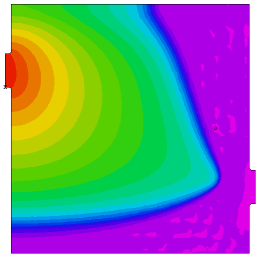﻿ Sample Problems > Applications > Fluids > black_oil

# black_oil

Navigation:  Sample Problems > Applications > Fluids >

# black_oil{  BLACK_OIL.PDE

This example considers the transport of oil and water in soil.

The model is given in Gelinas, et al, "Adaptive Forward-Inverse Modeling

of Reservoir Fluids Away from Wellbores",  (Lawrence Livermore National

Laboratory report UCRL-ID-126377) and in Saad & Zhang, " Adaptive Mesh for

Two-Phase Flow in Porous Media" (in Recent Advances in Problems of Flow and

Transport in Porous Media, Crolet and El Hatri, eds., Kluwer Academic Publishers,

Boston, 1998).

The saturation of water is represented by S, with the saturation of oil defined

as 1-S.  The relative permeabilities of water and oil are assumed to be S^2 and

(1-S)^2, respecitvely.  The total mobility M is defined as

M = S^2/muw + (1-S)^2/muo,

where muw and muo are the viscosities of water and oil.

The total velocity, V, and the fractional flux, f, are defined as

f = [S^2/muw]/M,

where K represents the saturation-independent permeability coefficient, and

P is the pressure, assuming capillary to be zero and oil and water pressures

equal.

If the porosity Phi is taken as constant and gravity effects are negligible, the

PDE's governing the system reduce to

Phi*dt(S) + div(V*f) = 0

div(V) = 0.

Here we study the flow through a 30-meter box with an inlet pipe in the upper

left and   an outlet pipe in the lower right.  The box is initially filled with oil,

and water is pumped into the inlet pipe at a constant pressure.  Time is measured

in seconds.

--  Submitted by Said Doss, Lawrence Livermore National Laboratory.

}

TITLE 'Black Oil Model'

SELECT

smoothinit   { Smooth the initial conditions a little, to minimize

the time wasted tracking the initial discontinuity }

VARIABLES

s(1), p         { Saturation and Pressure }

DEFINITIONS

muo = 4.e-3               { oil viscosity }

muw = 1.e-3               { water viscosity }

K = 1.e-12               { Saturation-independent permeability coefficient }

Pin = 1.5e6               { Inlet pressure }

Pout = 1.e6               { Outlet pressure }

M = S^2/muw + (1-S)^2/muo { Total mobility }

f = S^2/muw/M             { Fractional flux }

krw = S^2/muw             { Relative permeability of water }

phi =.206                 { porosity }

xmax = 30                 { Box dimensions }

ymax = xmax

out_ctr = 8

tfrac = 2*out_ctr

diam = 2

in_ctr = ymax-out_ctr

pipe = 2*rad         {an extended inlet and outlet pipe}

epsvisc = 1.e-6       { A little artificial diffusion helps smooth the solution }

sint = integral(s)   { the total extraction integral }

hour = 60*60

day = hour*24         { seconds per day }

INITIAL VALUES

s = max(0,-x/pipe )         { start with all oil , but ramp the value in the inlet pipe to speed startup }

p = Pin + (Pout-Pin)*x/xmax { start with a rough approximation to the pressure }

EQUATIONS

 BOUNDARIES   REGION 1     { fillet the input pipe, and define        no-flow boundaries of the box }     start(-pipe,in_ctr-diam)       natural(p)=0 natural(s) = 0       line to (0,in_ctr-diam) fillet(rad)       line to (0,0) to (xmax,0)             to (xmax,out_ctr-diam) fillet(rad)       line to (xmax+pipe,out_ctr-diam)         { set constant outlet pressure, and          "tautological" saturation flux }       value(p) = Pout       natural(s) =  -K*krw*dx(p)       line to (xmax+pipe,out_ctr+diam)         { reset no-flow box boundaries }       natural(p)=0 natural(s)=0       line to (xmax,out_ctr+diam) fillet(rad)       line to (xmax,ymax) to (0,ymax)             to (0,in_ctr+diam) fillet(rad)       line to (-pipe,in_ctr+diam)         { set constant inlet pressure and saturation }       value(p) = Pin   value(s) = 1       line to close   TIME   0 to 120*day by 10MONITORS

for cycle=5

contour(s) as "Saturation" range(0,1)

contour(s) zoom(xmax-tfrac+pipe,0, tfrac,tfrac) as "Outflow Saturation"

range(0,1)

contour(p) as "Pressure"

PLOTS

for t = day by day to 20*day

by 10*day to 120*day

grid(x,y)

contour(s) as "Saturation" range(0,1) painted

surface(s) as "Saturation" range(0,1) painted viewpoint(60,-120,30)

contour(s) zoom(xmax-tfrac+pipe,0,  tfrac,tfrac) as "Outflow Saturation"

range(0,1)  painted

contour(p) as "Pressure" painted